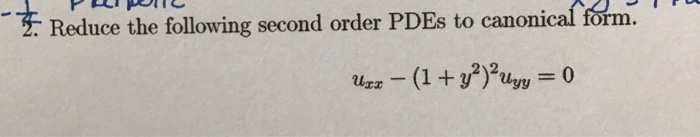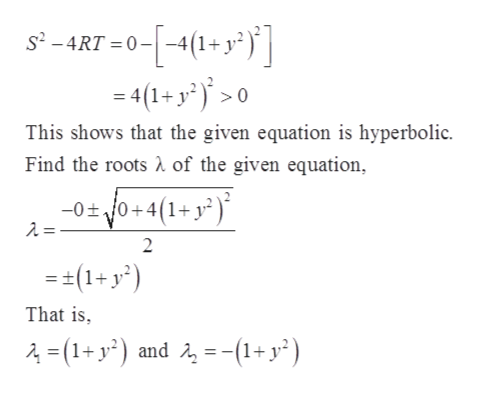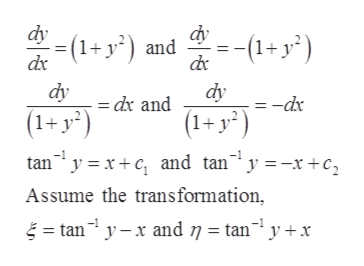# 2. Reduce the following second order PDES to canonical form.-(1+y2u= 0

Question
21 viewshelp_outlineImage Transcriptionclose2. Reduce the following second order PDES to canonical form. -(1+y2u= 0 fullscreen
check_circle

Step 1

The given differential equation,

Step 2

Check the given equation is hyperbolic, parabolic or elliptic.help_outlineImage Transcriptioncloses2-4RT 0--4(1+ y?) 4(1-y)> This shows that the given equation is hyperbolic. of the given equation Find the roots -0 0+4(1+y2) 2 =(1+y2) That is 4-(1+y and 1+y?) fullscreen
Step 3

Find the characteris...help_outlineImage Transcriptionclose--(1+y) (1+y2) and dx dy dy =-dx dx and (1+y2) (1+ y? tan yxc and tan" y =-x +c2 Assume the transformation tan yand 7 = tan y+x fullscreen

### Want to see the full answer?

See Solution

#### Want to see this answer and more?

Solutions are written by subject experts who are available 24/7. Questions are typically answered within 1 hour.*

See Solution
*Response times may vary by subject and question.
Tagged in

### Math